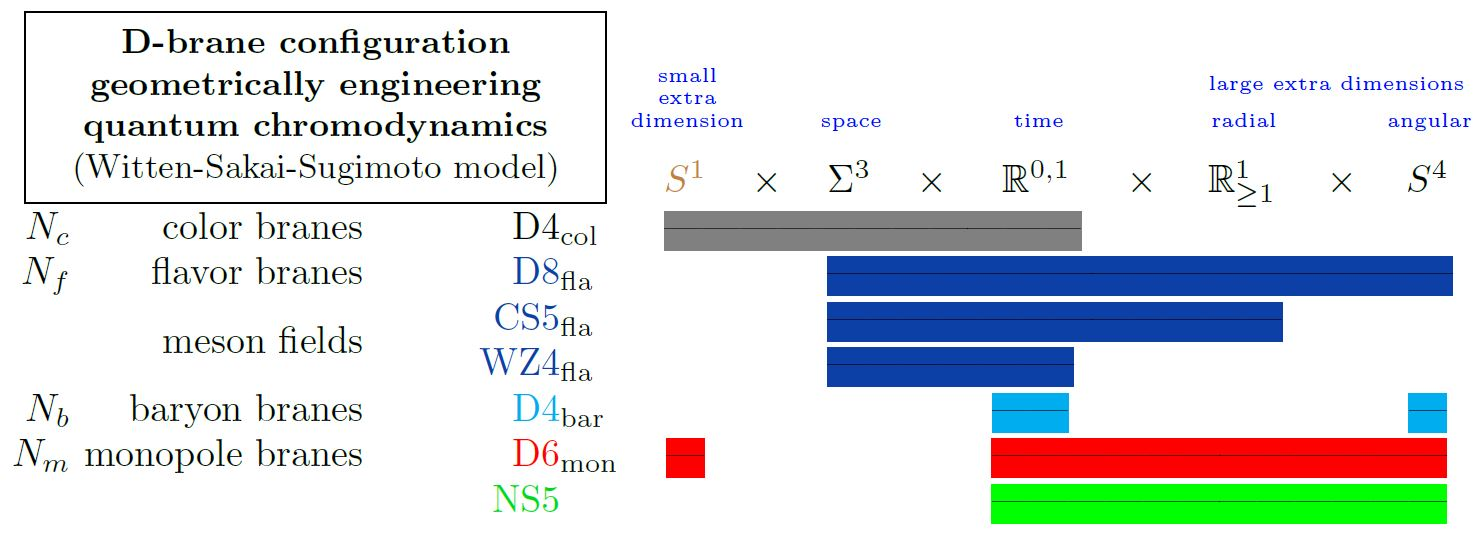# nLab nuclear matrix model

Contents

### Context

#### Fields and quanta

fields and particles in particle physics

and in the standard model of particle physics:

force field gauge bosons

scalar bosons

flavors of fundamental fermions in the
standard model of particle physics:
generation of fermions1st generation2nd generation3d generation
quarks ($q$)
up-typeup quark ($u$)charm quark ($c$)top quark ($t$)
down-typedown quark ($d$)strange quark ($s$)bottom quark ($b$)
leptons
chargedelectronmuontauon
neutralelectron neutrinomuon neutrinotau neutrino
bound states:
mesonslight mesons:
pion ($u d$)
ρ-meson ($u d$)
ω-meson ($u d$)
f1-meson
a1-meson
strange-mesons:
ϕ-meson ($s \bar s$),
kaon, K*-meson ($u s$, $d s$)
eta-meson ($u u + d d + s s$)

charmed heavy mesons:
D-meson ($u c$, $d c$, $s c$)
J/ψ-meson ($c \bar c$)
bottom heavy mesons:
B-meson ($q b$)
ϒ-meson ($b \bar b$)
baryonsnucleons:
proton $(u u d)$
neutron $(u d d)$

(also: antiparticles)

effective particles

hadrons (bound states of the above quarks)

solitons

in grand unified theory

minimally extended supersymmetric standard model

superpartners

bosinos:

dark matter candidates

Exotica

auxiliary fields

#### Duality in string theory

duality in string theory

general mechanisms

string-fivebrane duality

string-string dualities

M-theory

F-theory

string-QFT duality

QFT-QFT duality:

# Contents

## Idea

The nuclear matrix model (Hashimoto-Iizuka-Yi 10, Hashimoto-Matsuo-Morita 19) is a matrix model for baryons/nucleons in nuclear physics.

The model proceeds from the Witten-Sakai-Sugimoto model for QCD realized on intersecting D-branes, where baryons are embodied by wrapped D4-branes (“Witten’s baryon vertex”) on flavour D8-branes (D4-D8 brane bound states).(graphics from Sati-Schreiber 19c)

Thus, encoding the D-brane dynamics of these D4-branes in a matrix model along the lines of the BFSS matrix model/BMN matrix model (for D0-branes) and the IKKT matrix model (for D(-1)-branes) leads to a matrix model for baryons and hence, potentially, for nucleons.

Formulation of the model:

Review:

Further development:

Computation of nuclear binding energies in the model: